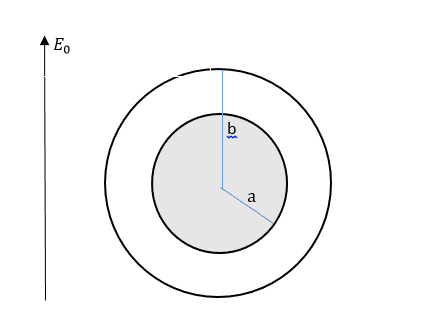# Wire surrounded by a linear dielectric in a uniform E field

physicsisfun0

## Homework StatementWe have an uncharged, conducting wire with radius a. We surround it by a linear dielectric material, εr, which goes out to radius b. We place this in an external electric field, Eo.

## Homework Equations

We have electric potential inside (a < s < b)
Vinbetween=Acosφ + (B/s)cosφ
and outside (s > b)
Voutside=-Eoscosφ + (D/s) cosφ

Our boundary conditions are:
Vinbetween=Voutside
εrEsinrEsout
when s = a, V = 0
when s = b, V = -Eoscosφ

## The Attempt at a Solution

I have solved for the constant and got:
A = - B/a2
B = (-Eob2 + D)/(b2(a-2 + a-2)
D = -((εrb2Eo + εrBEo + (Eo/a2) + (Eo/a2))b)/(b(a-2 + b-2 - εr + εrb)

Is this correct? Once I know these, I can find electric potential and electric field. From there, how would I go about solving for surface density on the dielectric of a bound and free charge, when s=a and s=b.

#### Attachments

It is not clear that you have applied the boundary conditions correctly. Presumably there is vacuum for s > b therefore there ought to be terms with ε0 coming from the continuity of the normal component of ##\vec D## across the boundary, $$\epsilon_r \frac{\partial V_I}{\partial s}=\epsilon_0 \frac{\partial V_{II}}{\partial s}$$ Also the sum in the expression for coefficient D (Eo/a2))b)/(b(a-2 + b-2 - εr + εrb) is dimensionally inconsistent.